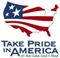Scientific Investigations Report 2008–5093

U.S. GEOLOGICAL SURVEY
Scientific Investigations Report 2008–5093

## Conversion Factors, Datums, and Abbreviations and Acronyms

### Inch/Pound to SI

Multiply By To obtain
cubic foot per second (ft3/s) 0.02832 cubic meter per second
cubic yard (yd3) 0.7646 cubic meter
foot (ft) 0.3048 meter
foot per foot (ft/ft) 1.0 meter per meter
foot per second (ft/s) 0.3048 meter per second
foot per squared second (ft/s2) 0.305 meter per squared second
inch (in.) 2.54 centimeter
inch (in.) 25.4 millimeter
mile (mi) 1.609 kilometer
Pascal (Pa) 1.0 Newtons per square meter
pound per cubic foot (lb/ft3) 0.00000624 milligram per liter
pound per foot-squared second [lb/(ft-s2)] 1.488 Newtons per square meter
square mile (mi2) 2.590 square kilometer
ton 907.185 kilogram
ton 0.907 metric ton
ton per day (ton/d) 0.01050 kilogram per second

### SI to Inch/Pound

Multiply By To obtain
gram (g) 0.03527 ounce, avoirdupois
kilogram per cubic meter (kg/m3) 0.063 pound per cubic feet
kilogram per meter per squared second [kg/(m-s2)] 1.0 Newtons per squared meter
meter (m) 3.281 foot
meter per second (m/s) 3.281 foot per second
meter per second squared (m/s2) foot per second squared
millimeter (mm) 0.039 inch
Newtons per square meter (N/m2) 0.6719 pound per foot squared per second
Newtons per square meter (N/m2) 1.0 Pascal (Pa)
square meter per second (m2/s) 1.076 square foot per second

Temperature in degrees Celsius (°C) may be converted to degrees Fahrenheit (°F) as follows:

°F=(1.8×°C)+32.

Concentrations of suspended sediments in water are given in milligrams per liter (mg/L).

### Datums

Vertical coordinate information refers to the North American Vertical Datum of 1988 (NAVD 88).

Horizontal coordinate information refers to the North American Datum of 1983 (NAD 83).

National Geodetic Vertical Datum of 1929 (NGVD 29) refers to a geodetic datum derived from a general adjustment of the first-order level nets of both the United States and Canada.

Lake datum is vertical datum used by the AVISTA Corporation and others in the Coeur d’Alene area. To obtain elevations in NAVD 88, add 0.80 ft to lake datum elevations.

### Abbreviations and acronyms

Abbreviations and acronyms Meaning
1D one-dimensional
2D two-dimensional
2.5D two and one-half dimensional
FEMA Federal Emergency Management Agency
GPS Global Positioning System
LIDAR Light Detection and Ranging
M1 curve backwater curve
M2 curve free-flowing water curve (above critical depth)
MD maximum difference
MD_SWMS Multi-Dimensional Surface Water Modeling System
NAIP National Agriculture Imagery Program
NF North Fork
RM river mile
SF South Fork
SI International System
USGS U.S. Geological Survey
WY water yearU.S. Department of the Interior | U.S. Geological Survey